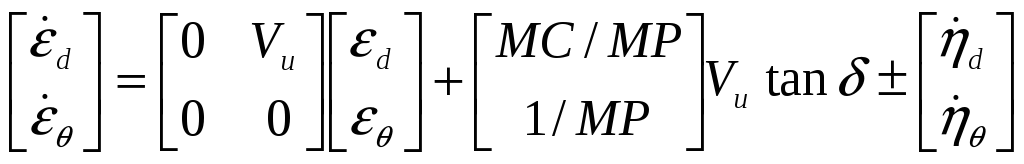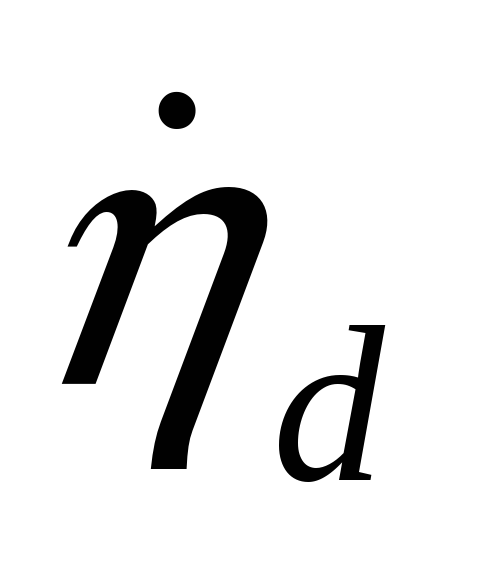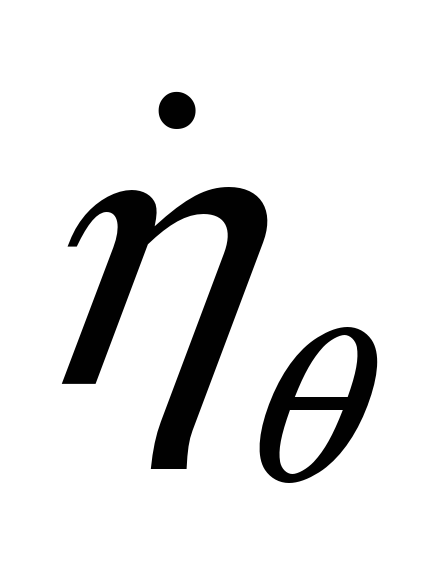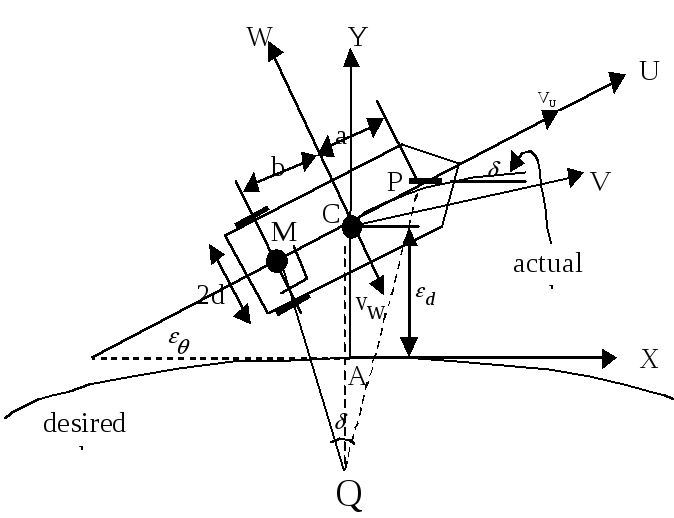# Soft Computing-based Design and Control for Mobile Robot Path Tracking

 Page 1/4 Date 05.05.2018 Size 371.02 Kb.
1   2   3   4

Soft Computing-based Design and Control for

Mobile Robot Path Tracking

Abdollah Homaifar

NASA ACE Center

Department of Electrical Engineering

## North Carolina A&T State University

Greensboro, NC 27411

Daryl Battle

Lucent Technologies

Whippany, NJ 07981

Edward Tunstel

Jet Propulsion Laboratory

California Institute of Technology

### Abstract

A variety of evolutionary algorithms, operating according to Darwinian concepts, have been proposed to approximately solve problems of common engineering applications. Increasingly common applications involve automatic learning of nonlinear mappings that govern the behavior of control systems. In many cases where robot control is of primary concern, the systems used to demonstrate the effectiveness of evolutionary algorithms often do not represent practical robotic systems. In this paper, genetic programming (GP) is the evolutionary strategy of interest. It is applied to learn fuzzy control rules for a practical autonomous vehicle steering control problem, namely, path tracking. GP handles the simultaneous evolution of membership functions and rule bases for the fuzzy path tracker. As a matter of practicality, robustness of the genetically evolved fuzzy controller is demonstrated by examining the effects of sensor measurement noise and an increase in the robot's nominal forward velocity.

1. Introduction

In recent years, increased efforts have been centered on developing intelligent control systems that can perform effectively in real-time. These include the development of non-analytical methods of soft computing such as evolutionary computation and fuzzy logic. These methods have proven to be effective in designing intelligent control systems and handling real-time uncertainty, respectively [1, 2]. In this paper, our efforts are focused on combining these paradigms to develop path tracking controllers for autonomous vehicles such as mobile robots and automated guided vehicles (AGVs). Specifically, we employ genetic programming (GP) for off-line learning of path tracking rules to be implemented in a fuzzy logic controller.

Genetic programming  has recently been demonstrated to be a viable approach to learning fuzzy logic rules for mobile robot control and navigation [4, 5]. Herein, we address the simultaneous design of fuzzy logic controllers (FLCs) using GP, i.e. evolution of both the input membership functions and the rule base. In addition, we extend the evolutionary influence of GP by incorporating the random selection of fuzzy logic connectives (t-norms) into the learning process. Finally, we examine the robustness of the evolved controllers by corrupting sensory data used by the path following robot, and by increasing the nominal forward velocity of the vehicle. This provides an indication of how well GP can evolve practical solutions that also retain the tolerance of imprecision and uncertainty characteristic of FLCs.

2. Overviews of Fuzzy Control and GP
A FLC is an intelligent control system that smoothly interpolates between rules, i.e. rules fire to continuous degrees and the multiple resultant actions are combined into an interpolated result. A fuzzy set may be represented by a mathematical formulation known as a membership function. That is, associated with a given linguistic variable (e.g. speed) are linguistic values or fuzzy subsets (e.g. slow, fast, etc.) expressed as membership functions which represent uncertainty, vagueness, or imprecision in values of the linguistic variable. These functions assign a numerical degree of membership to a crisp (precise) number. More precisely, over a given universe of discourse (relevant numerical range) X, the membership function of a fuzzy set, denoted by (x), maps elements xX into a numerical value in the closed unit interval, i.e. (x): X [0, 1].

Implementation of a fuzzy controller requires assigning membership functions for inputs and outputs. Inputs to a fuzzy controller are usually measured variables, associated with the state of the controlled plant, that are fuzzified (assigned membership values) before being processed by an inference engine. The heart of the controller inference engine is a set of if-then rules whose antecedents and consequences are made up of linguistic variables and associated fuzzy membership functions. Consequences from fired rules are numerically aggregated by fuzzy set union and then collapsed (defuzzified) to yield a single crisp output as the control signal for the plant. For detailed introductions to fuzzy control, fuzzy set operations, and concepts of fuzzification, inference, aggregation, and defuzzification see one of [2, 6].

In the GP paradigm, a population is comprised of computer programs or procedures (individuals) that are candidate solutions to a particular problem. These individuals participate in a simulated evolution process wherein the population evolves over time in response to selective pressure induced by the relative fitness of individuals in the problem domain. In our approach, each program executes condition-action statements, which collectively serve as a rule base to be embedded in a fuzzy controller. To preserve diversity among populations and vital genetic information among individuals, genetic operators are applied to create new individuals for succeeding generations. When the algorithm finally converges or satisfies its termination criteria, it is anticipated that the best (most fit) individual will be representative of an optimum or near optimum solution.

In the next section, we introduce the autonomous vehicle control problem, followed by discussion of FLC design issues to be considered when employing GP.

1. Mobile Robot Path Tracking Problem

The control problem examined in this paper is a path tracking problem, which was formulated by Hemami et al [7, 8] for a class of low speed (less than 2 m/s) tricycle-model vehicles. Essentially, the control objective is to successfully navigate a mobile robot or AGV along a desired path in a two-dimensional environment. We wish to automatically design a fuzzy controller that will achieve this objective. The inputs consist of a measurable position error, d, and a measurable orientation error, , associated with path following in the plane (see Figure 1). The output is the steering angle, , which is the corrective control action that would cause the errors to approach zero and, thus, force the robot to follow the desired path.

The position error is taken as the deviation of the center of gravity, C, or any other desired point of the robot from the nearest point on the path. The orientation error is the angular deviation of the robot from the tangent of the desired path. Hemami et al derived a state-space kinematic model for this robot where the state vector was comprised of the pose errors described. The reader is referred to either of  or  for details of the model derivation, which culminates in the following:(1)
where Vu is forward linear velocity of the robot, andandare rates of change of the effects of path curvature. In  it is concluded (based on dynamic analysis of the same vehicle) that for small steering angle, (tan ), Equation (1) approximates the slow dynamics of the vehicle when its forward velocity is low. For simulations presented later, we have simplified the robot kinematic model by taking this small steering angle approximation into account. Furthermore, we apply the controller to straight-line path following and, therefore, neglect the model effects of path curvature. Such a simplification does not preclude autonomous tracking of reasonably complicated paths since multi-segment paths can be defined to be piecewise linear.Figure 1. Tracking control and error variables.

For our application, we assume that the robot has dead-reckoning/odometry sensors that provide access to the error states at all times, or permit calculations thereof. This sensory input data is then mapped to control outputs according to the desired control policy. In path following simulations the state vector of the kinematic model is updated using the well-known fourth-order Runge-Kutta numerical integration method.